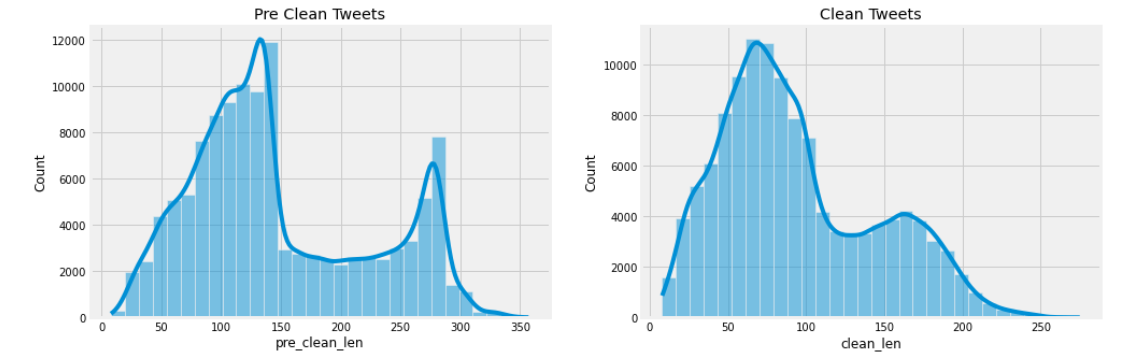# Sentiment Analysis of Tweets About Gabapentinoids

`#Creating dataframe called 'data' and storing the tweets 'Pregabalin' written in English.data = pd.DataFrame(itertools.islice(sntwitter.TwitterSearchScraper(    "pregabalin lang:en").get_items(), 50000))end_time = datetime.now()`
`df = (    df    .assign(sentiment = lambda x: x['content'].apply(lambda s: sentiment_classifier(s)))    .assign(         label = lambda x: x['sentiment'].apply(lambda s: (s['label'])),         score = lambda x: x['sentiment'].apply(lambda s: (s['score']))    ))`
`from afinn import Afinnafn = Afinn(emoticons=True)scores = [afn.score(content) for content in df.content]sentiment = ['positive' if score > 0 else 'negative' if score < 0 else 'neutral' for score in scores]df['af_scores'] = scoresdf['af_sentiment'] = sentiment`
• Extract the lengths of the tweets for posterior analysis
• Change the ‘date’ column to DateTime format and set it as the index.
• Lowercasing
• Keep only the tweets that contain the terms ‘gabapentinoids’, ‘gabapentin’, ‘neurontin’, ‘lyrica’, ‘pregabalin’, ‘gralise’, and ‘horizant’.
• Extract hashtags for posterior analysis.
• Removing URLs
• Replacing emoticons
• Removing non-alphanumeric characters
• Removing consecutive letters.
• Removing strings with just one alphanumeric character
• Removing stopwords
• Lemmatizing
• Length of the tweets before and after cleaning:Tweet length before and after cleaning
• Let’s see what the yearly boxplots look like:
• Yearly distribution of tweets:
• Tweets with more likes:
• Tweets with more retweets:
• Tweets with more replies:
• Most common unigrams:
• Most frequent combinations of two words or bigrams:
• Most common combinations of three words, or trigrams
• Most common hashtags:

--

--